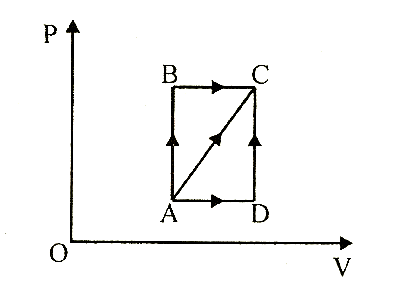# A thermodynamic process is shown in the figure. The pressures and volumes corresponding to some points in the figure arePA = 3 × 104 Pa  VA = 2 × 10-3 m3 PB = 8 × 104 Pa VD = 5 × 10-3m3.In process AB, 600 J of heat is added to the system and in process BC, 200 J of heat is added to the system. The change in internal energy of the system in process AC would be            (a)    560 J         (b)    800 J         (c)    600 J         (d)    640 J

10 years ago

(a)

Since AB is an isochoric process, so, no work is done. BC is isobaric process,

\ W = PB × (VD - VA) = 240 J

Therefore, DQ = 600 + 200 = 800 J

Using DQ = DU + DW

Þ DU = DQ - DW = 800-240 = 560 J

2 years ago
Since ab is an isochoric process -volume constant
Bc is isobaric process -pressure constant
DeltaQ =600j+200j
Work is 240j
DeltaQ=deltaU+ work
Work=800-240
560j